## Two step word problems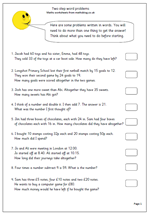Here we have a page of word problems which require more than one step in order to answer them. Children find this kind of two step word problem question much harder than when there is just one step involved in answering a question.

Often these questions can be done in several different ways. For example, the first question;

‘Jacob had 60 toys and his sister, Emma, had 48 toys. They sold 33 of the toys at a car boot sale. How many do they have left?’

The obvious way to do this is to add 60 and 48, making 108 and then subtracting 33 to leave 75.

However, it is just as valid, and maybe easier, to subtract 33 from 48 which is 15 and then add this on to the 60, to make 75. The actual numbers involved here are easier to deal with.

It is well worth keeping an open mind on how these types of questions should be answered and discuss with your children the best ways of approaching them.

This page can be found in the Year 4 resources under the Using and Applying Maths category.

2 step word problems

## Resource of the Week: Year 2 block graphs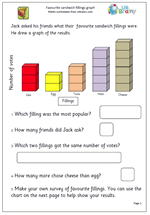It is in year 2 that children really get to grips with simple block graphs and here is one of the superb sets of year 2 maths worksheets which URBrainy.com have allowed me to publish. There are two aspects to block graphs. Firstly, interpreting a graph and understanding what it shows. Secondly, collecting suitable data to create a block graph.

This page is suitable for 6/7 year old children and looks at the favourite sandwich fillings of a class:- always a popular subject.

The first page involves interpreting the results of the graph, whilst the second page is a template which can be printed and used by the child to show the results of their own survey. It is well worth going to URBrainy as a simple log in process allows access to all their resources for a week as well as printing up to ten sets of worksheets. If you are looking for full and detailed coverage of Key Stage 1 then I can highly recommend this.

Sandwich graph

## Year 3 mental arithmetic questions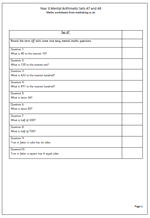This week we round off the term with some nice straightforward questions.

The first four questions in each set are about rounding to the nearest 10 or 100. Watch out for children who round to the nearest ten when they are meant to round to the nearest 100.

The next four questions look at doubling and halving 2-digit numbers and 3-digit multiples of 100. Doubling and halving are powerful mental arithmetic skills to possess and can help answer quite tricky questions easily, so it is well worth getting children to learn, off by heart, the doubles and halves of small numbers.

Finally, a couple of questions on the properties of squares and cubes. All quite straightforward!

Year 3_mental arithmetic_sets 47 and 48

## Easter maths worksheet: Co-ordinates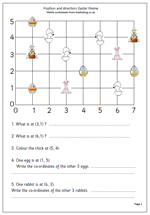This is a step up from the Easter maths worksheet published earlier in the week on Position and Direction, with several differences.

Firstly the axes are numbered rather than the spaces. This is an important step as the conventions of using co-ordinates come into play. it is important to show how co-ordinates are written:

e.g. (3, 1)

Brackets are always placed around the co-ordinates, with the numbers separated by a comma. The position (3, 1) means 3 along and 1 up, which in this case takes you to the pink rabbit (and not the rabbit holding the basket of eggs).

The grid can be used for further work: e.g.

1.ask how you can move along the lines to go from one point to another (2 along and 1 up)

2. Draw more features at the points and ask where they are positioned.

3. Ask child to draw an egg at a certain point etc.

This can be found in the Year 4 Shape category.

Thanks to urbrainy.com for allowing me to use it.

Easter coordinates

## Position and direction maths worksheet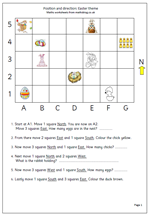Here we have a worksheet that takes an early look at position and direction on a grid. Firstly note that whilst the North arrow is showing the rest of the points of the compass are not showing, so it would be a good idea to go over these before attempting this page.

The second thing to note is that it is the squares rather than the lines which are numbered/lettered. The usual convention of reading along the horizontal axis and then the vertical axis applies.

The third thing to note is that each move is made from the position last reached and not reverting back to the start.

The theme is an Easter one, so March/April would be an ideal time for this.

Thanks to urbrainy.com for letting me use this page from their selection of Easter maths activities.

This worksheet can be found in the Year 3 Shape category.

Easter: position and direction

## Year 3 mental arithmetic: Sets 45 and 46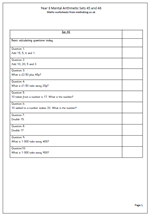As we approach the end of the Spring Term it’s time for another set of mental arithmetic questions. This week it is a mixed bag of calculating, including money.

The first two questions in each set look at adding 4 small numbers. The difficulty in doing this should not be underestimated as it is as much to do with memory as addition skills. This is a much easier question if children can see the numbers, either on the printed page or on a whiteboard.

The next two questions look at addition and subtraction of money. In both cases the pounds stay the same and the pence have to be added or subtracted.

Question 6 can cause difficulties. It asks, ’10 taken from a number is 17. What is the the number?’

Watch out for the child who hears the numbers and just subtracts 10 from 17!

Finally we have some doubling questions. By now children in Year 3 should know the double of small numbers up to ten and be able to work out the double of numbers in the teens. Eventually it is hoped they will know these off by heart.

Year 3 Mental arithmetic (sets 45 and 46)

## Subtraction of 3-digit numbers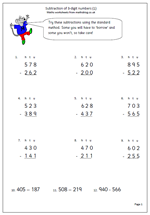Here is a maths worksheet that concentrates on practising subtraction of 3-digit numbers, using the standard, or efficient, written method.

There are several things to look out for when subtracting, including:

a. where each of the three digits on the bottom line are smaller than the 3 digits above them e.g.

987
654

This is the easiest type of question as there is no ‘borrowing’ or ‘adjusting.

b. Where the units are larger on the bottom line e.g.

456
327

Here an adjustment of ten has to be made from the tens column to the units column to carry out the subtraction.

c. Where the tens are larger on the bottom line e.g.

746
395

here an adjustment from the hundreds to the tens has to be made.

d. Where an adjustment from the tens has to be made, but there are no tens and the adjustment has to be made from the hundreds. e.g.

703

445

This is the hardest and one which many children will make a mistake.

For further details of these go to our Four Rules/Subtraction pages.

Subtraction of 3-digit numbers

## Resource of the Week: Simple Maths Games

Whilst most of the mathsblog site is about maths worksheets we do have a small selection of fun maths games for young children.

Listen under the Reception/Year 1 Maths Games there are over 20 simple maths games which younger children children may like to play and which will certanly help with counting, ordering and addition .

Here is one example to help with counting.

Help Ted colour his white balloons. Just by clicking in the balloons the colour will change.

One click will make a balloon go Red.

Two clicks will turn the balloon Blue.

Three clicks will change the colour to Gold.

Don’t forget to press Go when you think the colours are correct!

This is great practice at counting, why not have a go?

More games can be found in our Maths Games section.

## Year 3 Mental Arithmetic Worksheets: Sets 43 and 44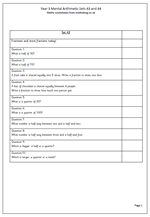This week’s mental arithmetic questions for Year 3 are all about fractions. The first couple of questions are simple, finding halves of multiples of 10. Most children learn the halves of even numbers up to 10 but find it harder to work out half of numbers such as 30 or 50, where this knowledge is not so useful.

The next two questions look at a different aspect of fractions; sharing one whole one into equal parts and naming the parts. Questions 5 and 6 look at finding a quarter of numbers.

Probably the hardest two questions involve counting up in quarters and finding a number half way between two others. Finding the number half way between three and a half and four is quite tricky and children need to have spent some time using number lines and counting in quarters to be successful with this.

Finally a couple of questions which test knowledge of the meaning of a fraction and that the larger the bottom number (denominator) the smaller the fraction will be (if the top number (numerator) is the same).

Year 3 mental arithmetic (sets 43 and 44)

## More on equivalent fractions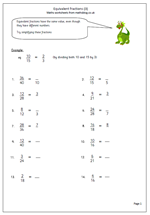Equivalent fractions are fractions which look different but have the same value. The easiest example of equivalent fractions is a half and two quarters. If I have half of a fruit cake that is the same as having two quarters of the same cake. (As long as I pick up all the crumbs from cutting it!)

Not all equivalent fractions are that easy to work out, but as a rule it can be said that:

If we multiply the top and bottom numbers in a fraction by the same value we will get an equivalent fraction.

The same can be said of division:

If we divide the top and bottom numbers of a fraction by the same value we will get an equivalent fraction.

Dividing is really useful as it ‘simplifies’ the fraction, often making it easier to read or to picture. For example 10/100 is equivalent to 1/10 as both top and bottom have been divided by 10.

Now, the fraction will only get simpler if we can successfully divide both top and bottom numbers without leaving a remainder! This is the key, and, as so often, a good knowledge of tables helps here.

This worksheet looks at simplifying fractions. As shown above, this is done by dividing the top and bottom of the fraction by the same number. This division process might need to be done more than once. For example: 8/12 can be simplified to 4/6. This in turn can be simplified to 2/3.

Equivalent fractions (pg 3)